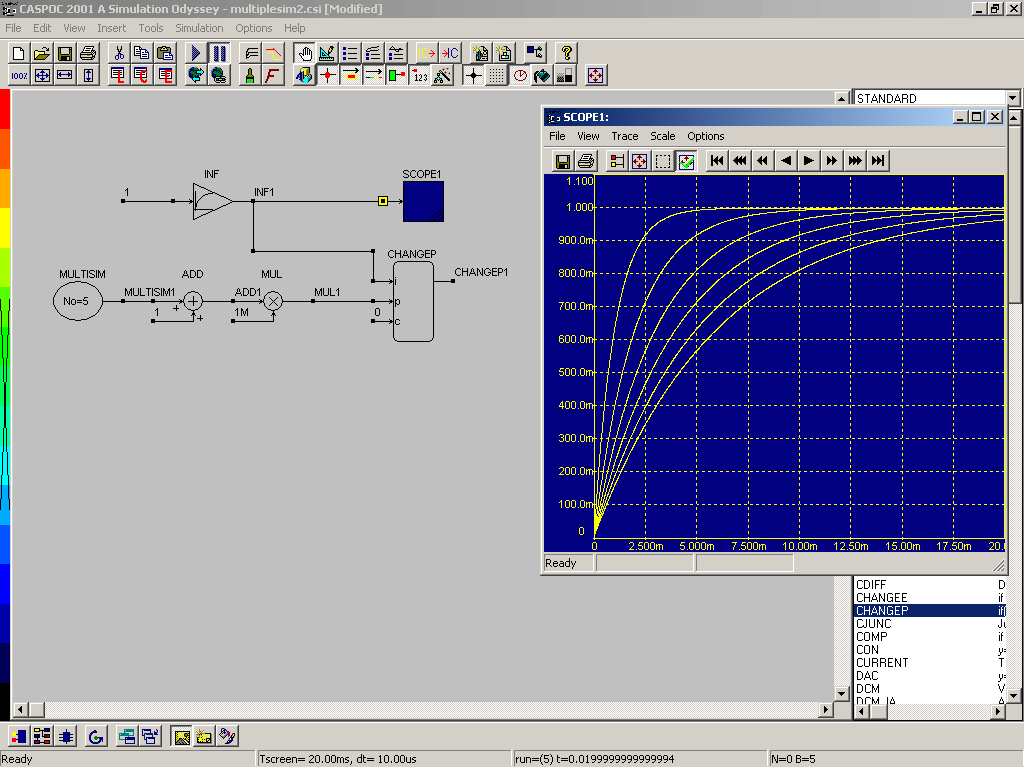• Introduction
• What is in this manual
• What is Caspoc
• User interface
• Introduction
• Starting
• Simulation
• Editing
• Viewing and printing
• Getting Started
• Basic editing
• Simulation in the time domain
• Basic User Interface Topics
• Editing
• Simulation
• Viewing
• Library
• Reports
• Project management
• Circuit and Block Diagram Components
• Introduction
• Cscript and user defined functions
• Component parameters
• Modeling Topics
• Introduction
• Power Electronics
• Semiconductors
• Electrical Machines
• Electrical drives
• Power Systems
• Mechanical Systems
• Thermal Systems
• Magnetic Circuits
• Green Energy
• Coupling to FEM
• Experimenter
• Analog hardware description language
• Embedded C code Export
• Coupling to Spice
• Small Signal Analysis
• Matlab coupling
• Tips and tricks
• Appendices

## Multiple simulations with parameter variations.

Suppose we want to make a simulation where we are interested in the dependence of the system on one or more parameters. Instead of changing the parameter and restarting the simulation manually, we use the "Multiple Simulation" option.

In a multiple simulation project, you use the block "Multisim" where the output indicates the number of the simulation.

If we want the multiple simulation to run 5 times, the output of the block "Multisim" will be equal to 0, 1, 2, .. 5, depending on the number of the simulation. Using the block-daigram, any parameter value can be constructed from the output of the block "Multisim".

In the dialog box "Multiple Simulation Parameters", which you open by selecting Simulation/Multiple Simulation Parameters you can specify the number of simulations.

The results from each simulation are stored in the "Scopes". In the following example, the transient response for various values of the resistor in the circuit is simulated. Create the RLC circuit using the components V=1volt, L=1mH, C=100uF, R=10ohm and one Scope. Place a scope at the output node of the RLC circuit as indicated in the figure below.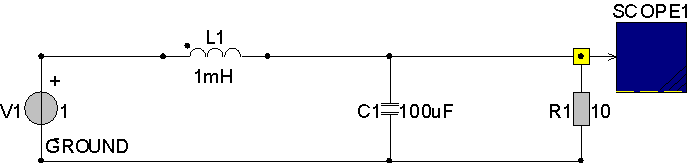Create a block-diagram with a "Multisim", "Mul" and "ChangeE" block.The "ChangeE" block changes the value of R1 during the simulation as long as the second input of he block is equal to 0. Set the second input of the "ChangeE" blobk to zero by selecting it with the right mouse button and typing 0.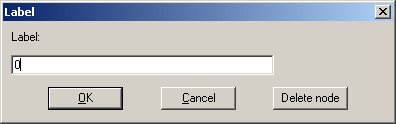Open the "ChangeE" blobk by clicking it with the right mouse button and type "R1" in the text box following Text1.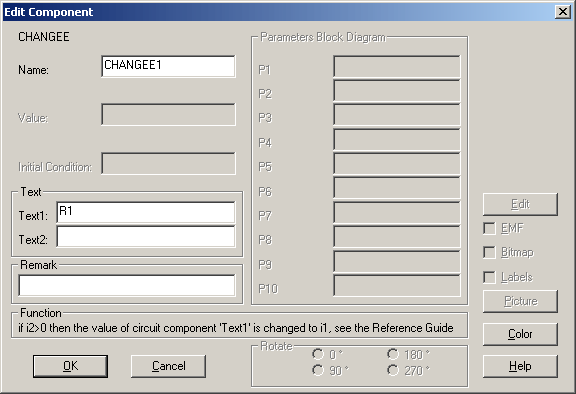Since the time response will be around 5ms, set Tscreen to 20ms and set dt to 100us. (Select Simulation/Simulation parameters to set the simulation parameters.To specify the number of multiple simulations, select Simulation/Multiple Simulation Parameters and enter 5 for the number of simulations.Instead of selecting the start button, select Simulation/Start Multiple Simulation After running 6 times the scope shows the responses for all 6 runs. Clicking on the scope with the right mouse button shows you the detailed responses.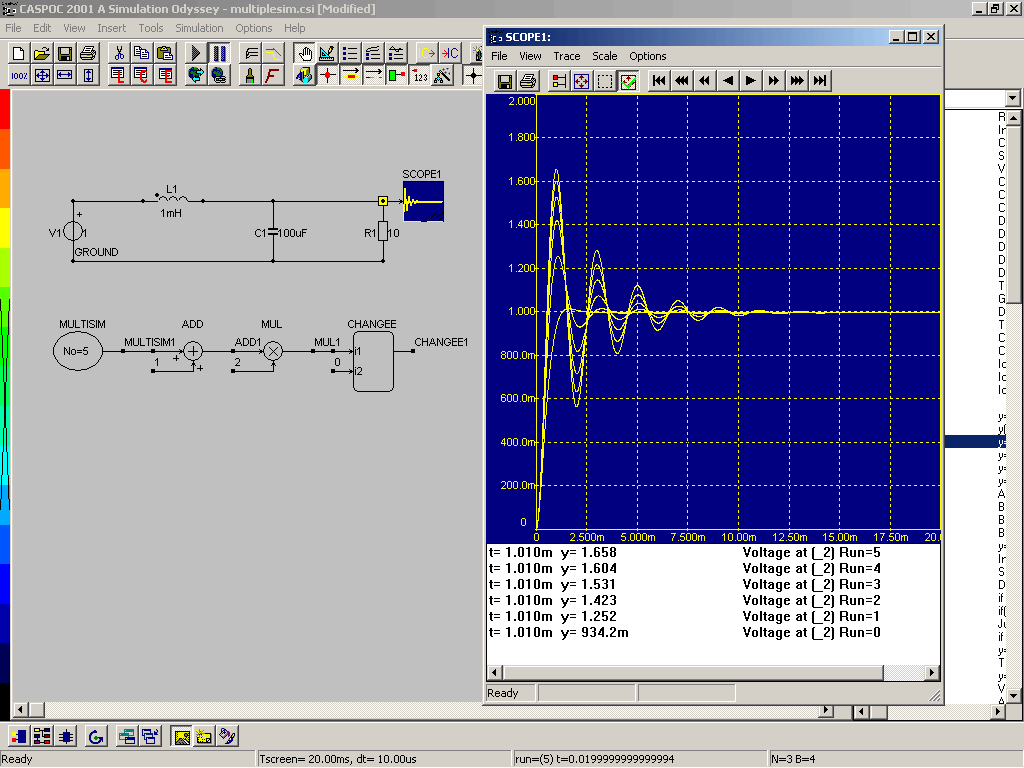You can use the arrow keys to step through the wave forms.

You can use many "Multisim" blocks in your simulation to control the value of components or parameters. For changing parameters in the block-diagram, use the block "ChangeP", where the first input of the block "ChangeP" is connected to the block where the parameter has to be changed.

Create the block-diagram as indicated in the figure below:The first input should be connected to the block in which we want to vhange the parameter. In this case we want to change the third parameter in the block "INF", which is the time constant tau of the first order differential equations modeled in the block "INF".The second input is the new value, which we want to place in the block "INF" using the "ChangeP" block. Set the third input to 0, so Caspoc will automatically determine when to change the parameter. To indicate which parameter to change in the block connected to the first input of the block "ChangeP", we have to specify the parameter p(n) in the block "ChangeP". Since we want to change the third parameter tau, specify 3.Start the multiple simulation and the 6 responses for tau=1ms..6ms are displayed in the scope window.